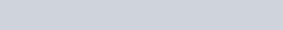Sodium stearate
Question:

Sodium stearateanionic surfactant which forms micelles in oil.

Choose the correct statement for it from the following :

1. It forms spherical micelles with $\mathrm{CH}_{3}\left(\mathrm{CH}_{2}\right)_{16}-$ group pointing towards the centre of sphere.

2. It forms non-spherical micelles with $-\mathrm{COO}^{-}$ group pointing outwards on the surface.

3. It forms spherical micelles with $\mathrm{CH}_{3}\left(\mathrm{CH}_{2}\right)_{16}-$ group pointing outwards on the surface of sphere

4. It forms non-spherical micelles with $\mathrm{CH}_{3}\left(\mathrm{CH}_{2}\right)_{16}$-group pointing towards the centre.

Correct Option: 1

Solution:

Forms spherical micelles with $\mathrm{CH}_{3}\left(\mathrm{CH}_{2}\right)_{16}$ group pointing towards the centre of sphere